# MathWorks Logo, Part One. Why Is It L Shaped?

MathWorks is the only company in the world whose logo satisfies a partial differential equation. Why is the region for this equation shaped like a capital letter L?

### Contents

#### Wave Equation

The wave equation describes how a disturbance travels through matter. If the units are chosen so that the wave propagation speed is equal to one, the amplitude of a wave satisfies $${{\partial^2 u} \over {\partial t^2}} = \triangle u$$ The $\triangle$ denotes Laplace's operator $$\triangle = {\partial^2 \over {\partial x^2}} + {\partial^2 \over {\partial y^2}}$$

#### Initial and Boundary Values

Geometry plays a crucial role here. Initial values of the amplitude and velocity of the wave are prescribed on a certain region. Values of the amplitude or its normal derivative are also prescribed on the boundary of the region. If the wave vanishes outside the region, these boundary values are zero.

#### Eigenvalues and Eigenfunctions

Separating out periodic time behavior leads to solutions of the form $$u(x,y,t) = \cos{(\sqrt{\lambda}\,t)} v(x,y)$$ The functions $v(x,y)$ also depend upon $\lambda$ and the region. They satisfy the differential equation $$\triangle v + \lambda v = 0$$ and are zero on the boundary of the region. The quantities $\lambda$ that lead to nonzero solutions are the eigenvalues, and the corresponding functions $v(x,y)$ are the eigenfunctions or modes. They are determined by the physical properties of the medium and the geometry of the region. The square roots of the eigenvalues are resonant frequencies. A periodic external driving force at one of these frequencies generates an unboundedly strong response in the medium. Any solution of the wave equation can be expressed as a linear combination of these eigenfunctions. The coefficients in the linear combination are obtained from the initial conditions.

#### One Dimension

In one dimension, the eigenvalues and eigenfunctions are easily determined. The simplest example is a violin string, held fixed at the ends of an interval. The eigenfunctions are trig functions. $$v_k(x) = \sin{(k x)}$$ If the length of the interval is $\pi$, the eigenvalues are determined by the boundary condition, $v_k(k \pi) = 0$. Hence, $k$ must be an integer and $$\lambda_k = k^2$$ If the initial condition is expanded in a Fourier sine series, $$u(x,0) = \sum_k a_k \sin{(k x)}$$ (And the initial velocity is zero), then the solution to the wave equation is $$u(x,t) = \sum_k a_k \cos{(\sqrt{\lambda_k}\,t)} v_k(x)$$ Here are graphs of the first nine eigenfunctions in one dimension. The corresponding eigenvalues are the squares of integers.
   eigenvals = (1:9).^2
plot_modes('1d')

eigenvals =
1     4     9    16    25    36    49    64    81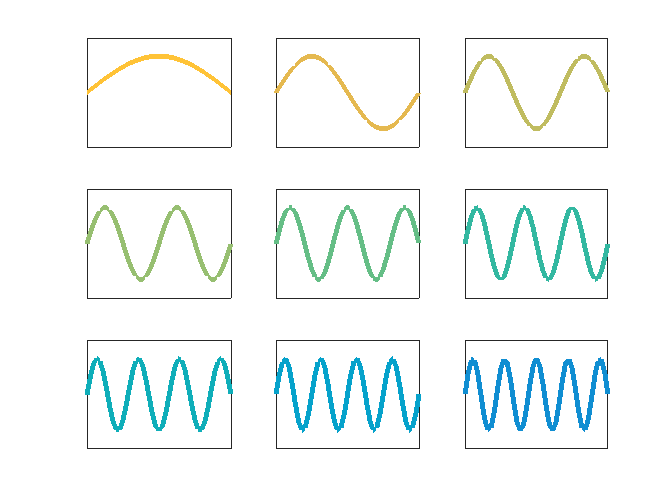#### A Square

The simplest region in two dimensions is a square. The eigenfunctions are again trig functions. $$v_{k,j}(x,y) = \sin{(k x)}\,\sin{(j y)}$$ If the sides have length $\pi$, the boundary conditions imply that $k$ and $j$ must be integers. Here are the first nine eigenvalues and eigenfunctions.
   [k,j] = meshgrid(1:3); e = k.^2+j.^2;  eigenvals = e(:)'
plot_modes('square')

eigenvals =
2     5    10     5     8    13    10    13    18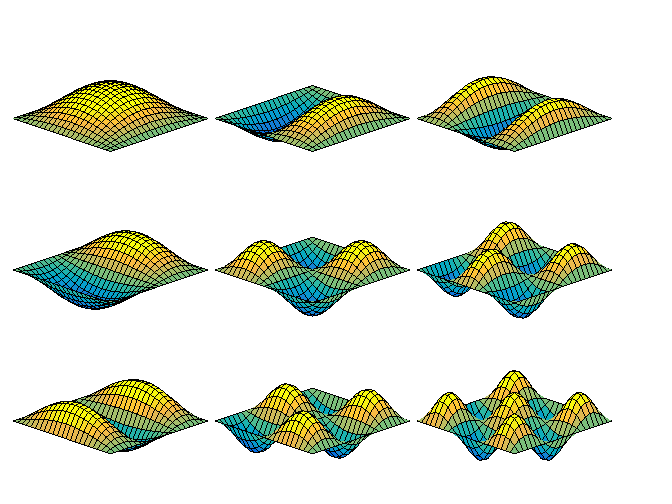#### A Circular Disc

If the region is a circular disc, we switch to polar coordinates, $r$ and $\theta$. Trig functions are replaced by Bessel functions. The eigenfunctions become $$v_{k,j}(r,\theta) = B_j(\mu_k r) \,\sin{(j \theta)}$$ where $B_j$ is the $j$ -th order Bessel function and $\mu_k = \sqrt{\lambda_k}$. To find the eigenvalues we need to have the eigenfunctions vanish on the boundary of the disc. If the radius is one, we require $$B_j(\mu_k) = 0$$ In other words, we need to compute zeros of Bessel functions. Here are the first nine eigenvalues and eigenfunctions of the circular disc. The violin string has become a tambourine.
   eigenvals = [bjzeros(0,3) bjzeros(1,3) bjzeros(2,3)].^2
plot_modes('disc')

eigenvals =
Columns 1 through 7
5.7832   30.4713   74.8870   14.6820   49.2185  103.4995   26.3746
Columns 8 through 9
70.8500  135.0207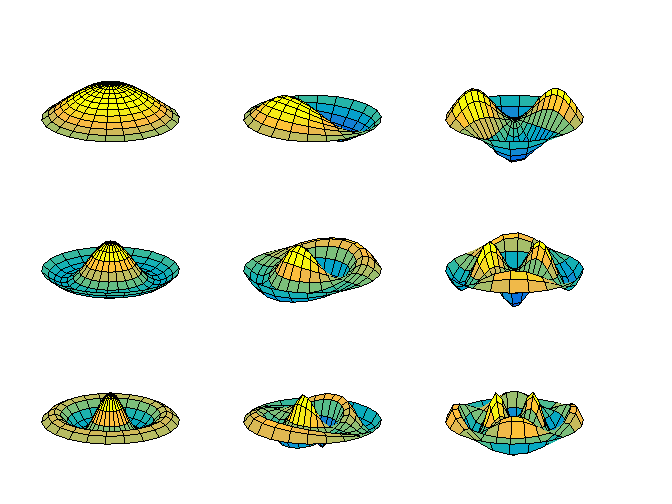#### A Circular Sector

Replace the full circular disc by a three-quarter circular sector. The angle at the origin is $270^\circ$ or $\frac{3}{2}\pi$ radians. We can make our eigenfunctions adapt to this angle. Take $$v_{k,j}(r,\theta) = B_{\alpha_j}(\mu_k r) \,\sin{(\alpha_j \theta)}$$ with $$\alpha_j = \frac{2}{3} j$$ and fractional order Bessel functions. The eigenfunctions satisfy their differential equation and also satisfy the boundary conditions on both sides of the angle. $$v_{k,j}(r,\theta) = 0$$ at $\theta = 0$ and at $\theta = \frac{3}{2}\pi$. By finding the zeros of the Bessel functions we can also have the eigenfunctions satisfy the boundary conditions on the outer circular portion of the boundary. Here are the first nine eigenvalues and eigenfunctions of the three-quarter circular sector.
   eigenvals = [bjzeros(2/3,3) bjzeros(4/3,3) bjzeros(6/3,3)].^2
plot_modes('sector')

eigenvals =
Columns 1 through 7
11.3947   42.6442   93.6362   18.2785   56.1131  113.6860   26.3746
Columns 8 through 9
70.8500  135.0207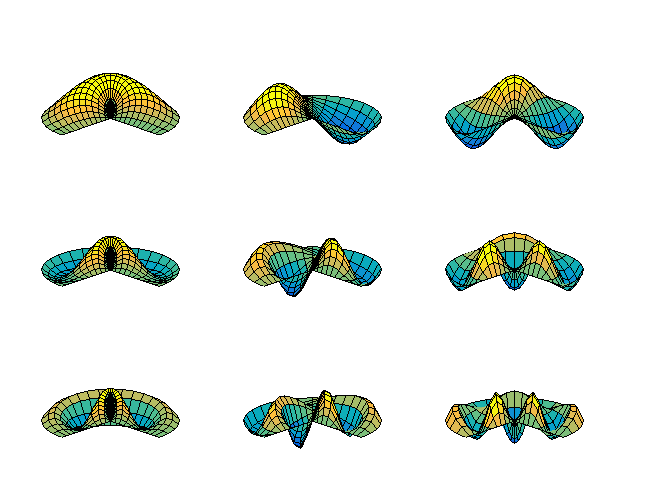These eigenfunctions have another important property. Most of them are singular; the derivatives of the fractional order Bessel functions are unbounded at the origin. You can see the black concentration of grid lines in the plots. If you tried to make a tambourine with this sector shape, it would rip at the sharp corner. This singular behavior is needed to model the solution to the wave equation on this region.

#### The L-shaped Membrane

For all the regions we have discussed so far it is possible to express the eigenvalues as zeros of analytic functions. For the interval and the square, the eigenvalues are integers, which are the zeros of $\sin{\pi x}$. For the circular disc and sector, the eigenvalues are zeros of Bessel functions. Once we have the eigenvalues, it is easy to compute the eigenfunctions using sines and Bessel functions. So, an L-shaped region formed from three unit squares is interesting for at least two reasons. It is the simplest geometry for which solutions to the wave equation cannot be expressed analytically; numerical computation is necessary. Furthermore, the 270 degree nonconvex corner causes a singularity in the solution. Mathematically, the gradient of the first eigenfunction is unbounded near the corner. Physically, a membrane stretched over such a region would rip at the corner. This singularity limits the accuracy of finite difference methods with uniform grids. I used the L-shaped region as the primary example in my doctoral thesis fifty years ago. MathWorks has adopted a modified surface plot of the first eigenfunction as the company logo. I am going to devote a series of blog posts to the L. Here are the first nine eigenvalues and eigenfunctions, computed by a function from Numerical Computing with MATLAB, which I will discuss in a future posting. Compare these eigenfunctions with the ones from the circular sector, which has the same reentrant corner and resulting singularity.
   for k = 1:9
[~,eigenvals(k)] = membranetx(k);
end
eigenvals
plot_modes('L')

eigenvals =
Columns 1 through 7
9.6397   15.1973   19.7392   29.5215   31.9126   41.4745   44.9485
Columns 8 through 9
49.3480   49.3480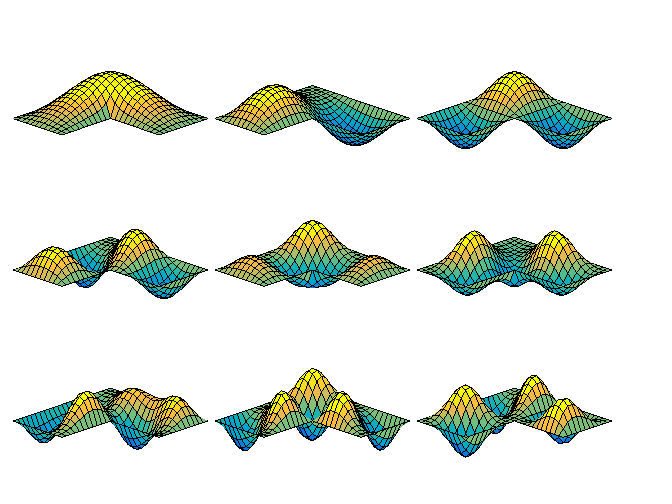#### Microwave Waveguide

Simple model problems involving waves on an L-shaped region include an L-shaped membrane, or L-shaped tambourine, and a beach towel blowing in the wind, constrained by a picnic basket on one fourth of the towel. A more practical example involves ridged microwave waveguides. One such device is a waveguide-to-coax adapter. The active region is the channel with the H-shaped cross section visible at the end of the adapter. The ridges increase the bandwidth of the guide at the expense of higher attenuation and lower power-handling capability. Symmetry of the H about the dotted lines shown in the contour plot of the electric field implies that only one quarter of the domain needs to be considered and that the resulting geometry is our L-shaped region. The boundary conditions are different than our membrane problem, but the differential equation and the solution techniques are the same. The photo is courtesy of Advanced Technical Materials, Inc. See their website, <http://atmmicrowave.com/>, for lots of devices like this.
   waveguide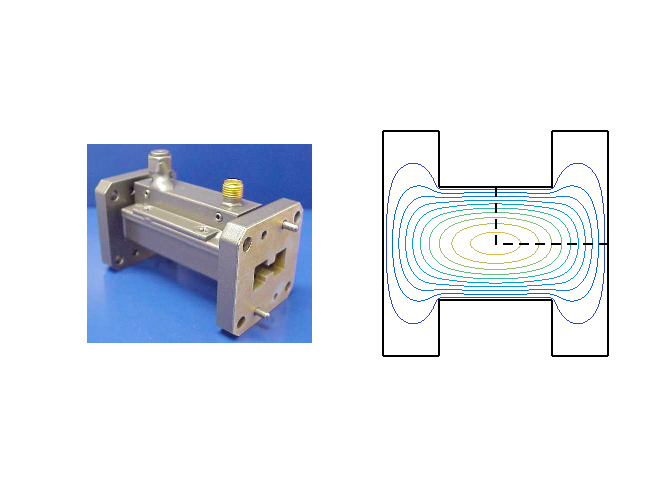Published with MATLAB® R2014b
|